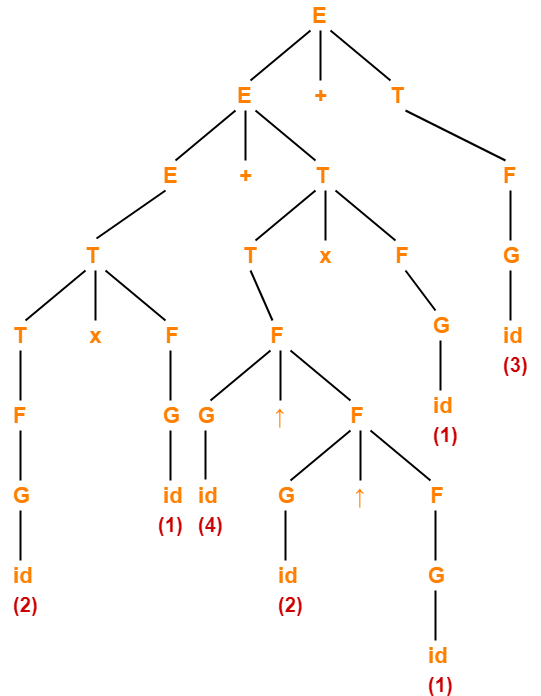Context Free Grammar | Context Free Language

Context Free Grammar-

A context Free Grammar (CFG) is a 4-tuple such that-

G = (V , T , P , S)

where-

• V = Finite non-empty set of variables / non-terminal symbols
• T = Finite set of terminal symbols
• P = Finite non-empty set of production rules of the form A → α where A ∈ V and α ∈ (V ∪ T)*
• S = Start symbol

Why Context Free Grammar Is Called So?

Context Free Grammar provides no mechanism to restrict the usage of the production rule A → α within some specific context unlike other types of grammars.

That is why it is called as “Context Free” Grammar.

Example-01:

Consider a grammar G = (V , T , P , S) where-

• V = { S }
• T = { a , b }
• P = { S → aSbS , S → bSaS , S → ∈ }
• S = { S }

• This grammar is an example of a context free grammar.
• It generates the strings having equal number of a’s and b’s.

Example-02:

Consider a grammar G = (V , T , P , S) where-

• V = { S }
• T = { ( , ) }
• P = { S → SS , S → (S) , S → ∈ }
• S = { S }

• This grammar is an example of a context free grammar.
• It generates the strings of balanced parenthesis.

Applications-

Context Free Grammar (CFG) is of great practical importance. It is used for following purposes-

• For defining programming languages
• For parsing the program by constructing syntax tree
• For translation of programming languages
• For describing arithmetic expressions
• For construction of compilers

Context Free Language-

 The language generated using Context Free Grammar is called as Context Free Language.

Properties-

• The context free languages are closed under union.
• The context free languages are closed under concatenation.
• The context free languages are closed under kleen closure.
• The context free languages are not closed under intersection and complement.
• The family of regular language is a proper subset of the family of context free language.
• Each Context Free Language is accepted by a Pushdown automaton.

Remember

If L1 and L2 are two context free languages, then-

• L1 ∪ L2 is also a context free language.
• L1.L2 is also a context free language.
• L1* and L2* are also context free languages.
• L1 ∩ L2 is not a context free language.
• L1′ and L2′ are not context free languages.

Ambiguity in Context Free Grammar-

A grammar is said to be ambiguous if for a given string generated by the grammar, there exists-

• more than one leftmost derivation
• or more than one rightmost derivation
• or more than one parse tree (or derivation tree).

To gain better understanding about Context Free Grammar,

Watch this Video Lecture

Next Article- Chomsky Normal Form

Get more notes and other study material of Theory of Automata and Computation.

Watch video lectures by visiting our YouTube channel LearnVidFun.

SummaryArticle Name
Context Free Grammar | Context Free Language
Description
Context Free Grammar- A context Free Grammar or CFG is a 4-tuple such that G = (V , T , P , S). Examples. Context Free Language is a language generated using Context Free Grammar. Applications of Context Free Grammar.
Author
Publisher Name
Gate Vidyalay
Publisher Logo